Get inspired by the success stories of our students in IIT JAM MS, ISI  MStat, CMI MSc DS.  Learn More

# ISI MStat PSB 2004 Problem 4 | Calculating probability using Uniform DistributionThis is a very beautiful sample problem from ISI MStat PSB 2004 Problem 4 based on finding the probability using Uniform distribution . Let's give it a try !!

## Problem- ISI MStat PSB 2004 Problem 4

Two policemen are sent to watch a road that is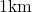long. Each of the two policemen is assigned a position on the road which is chosen according to a uniform distribution along the length of the road and independent of the other's position. Find the probability that the
policemen will be less than 1/4 kilometer apart when they reach their assigned posts.

### Prerequisites

Uniform Distribution

Basic geometry

## Solution :

Let X be the position of a policeman and Y be the position of another policeman on the road of 1km length .

As it is given that chosen according to a uniform distribution along the length of the road and independent of the other's position hence we can say that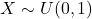and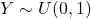and X,Y are independent .

Now we have to find the probability that the policemen will be less than 1/4 kilometer apart when they reach their assigned posts , which is

nothing but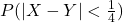.

So , let's calculate the probabilityhere some sort of geometry will help to calculate it easily !

In general we have 0<X<1 and 0<Y<1 and hence the total probability is the area of the square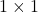And in favourable case we have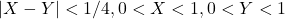. so, it's basically the area covered by ACBDEF = Area covered by square - area of the triangles BGD and AFH =-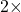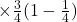=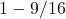.

Therefore## Food For Thought

Calculate the same under the condition that road is of length (b-a) , b>a and both are positive real number .

## Subscribe to Cheenta at Youtube

This is a very beautiful sample problem from ISI MStat PSB 2004 Problem 4 based on finding the probability using Uniform distribution . Let's give it a try !!

## Problem- ISI MStat PSB 2004 Problem 4

Two policemen are sent to watch a road that islong. Each of the two policemen is assigned a position on the road which is chosen according to a uniform distribution along the length of the road and independent of the other's position. Find the probability that the
policemen will be less than 1/4 kilometer apart when they reach their assigned posts.

### Prerequisites

Uniform Distribution

Basic geometry

## Solution :

Let X be the position of a policeman and Y be the position of another policeman on the road of 1km length .

As it is given that chosen according to a uniform distribution along the length of the road and independent of the other's position hence we can say thatandand X,Y are independent .

Now we have to find the probability that the policemen will be less than 1/4 kilometer apart when they reach their assigned posts , which is

nothing but.

So , let's calculate the probabilityhere some sort of geometry will help to calculate it easily !

In general we have 0<X<1 and 0<Y<1 and hence the total probability is the area of the squareAnd in favourable case we have. so, it's basically the area covered by ACBDEF = Area covered by square - area of the triangles BGD and AFH =-=.

Therefore## Food For Thought

Calculate the same under the condition that road is of length (b-a) , b>a and both are positive real number .

## Subscribe to Cheenta at Youtube

This site uses Akismet to reduce spam. Learn how your comment data is processed.

### Knowledge Partner